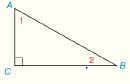Chapter 2.CT, Problem 18CT### Elementary Geometry for College St...

6th Edition
Daniel C. Alexander + 1 other
ISBN: 9781285195698

#### Solutions

Chapter
Section### Elementary Geometry for College St...

6th Edition
Daniel C. Alexander + 1 other
ISBN: 9781285195698
Textbook Problem
1 views

# In Exercises 16 and 18 , complete the missing statements or reasons for each proof. Given: In Δ A B C , m ∠ C = 90 °Prove ∠ 1 and ∠ 2 are complementary PROOF Statements Reasons 1. Δ A B C , m ∠ C = 90 ° 1.               _ 2. m ∠ 1 + m ∠ 2 + m ∠ C =             _ 2. The sum of ∠ s of a Δ is 180 ° . 3.                       _ 3. Substitution Property of Equality 4. m ∠ 1 + m ∠ 2 =                           _ 4. Subtraction Property of Equality. 5. ∠ 1 and ∠ 2 are complementary. 5.               _

To determine

To prove:

The given statement.

Explanation

Given:

The following figure shows the given diagram.

Given that, in ΔABC, mC=90°.

Concept:

(1). The angle must be complementary if sum of measure of angle is 90°.

(2). The angle must be complementary if sum of measure of angle is 90°.

Approach:

The completed proof is shown in the following table.

 PROOF Statements Reasons 1. ΔABC, m∠C=90° 1. Given 2.m∠1+m∠2+90°=180° 2. The sum of ∠s of a Δ is 180°. 3.m∠1+m∠2+m∠C=180° 3. Substitution Property of Equality 4. m∠1+m∠2=90° 4. Subtraction Property of Equality

### Still sussing out bartleby?

Check out a sample textbook solution.

See a sample solution

#### The Solution to Your Study Problems

Bartleby provides explanations to thousands of textbook problems written by our experts, many with advanced degrees!

Get Started

#### Find more solutions based on key concepts